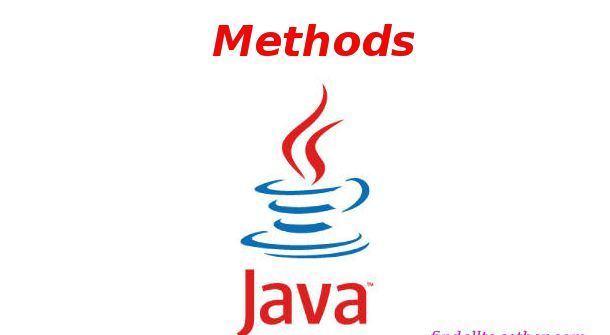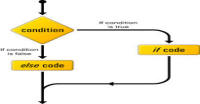# Methods in java

It is very excited tutorial for new programmers because after using number of built-in methods like print, println, random, etc. First summarize what we know about methods till now:

• Methods are collection of statements which perform some task.
• Method may have any argument (additional information).
• Method may return some value.

And one more thing we know about java that we can call static type objects directly using name of the class. Now we are making our own methods.

### Simple method

As you are already familiar with main method. The syntax of the method is:

```access_specifies type return_type method_name(argument_type argument_name,.....other_arguments....)
{
//code
}
```

Compare this syntax with main method.

access_specifier => public

type => static

return_type => void ( void means this method is not returning anything, discussed later in this tutorial)

method_name => main

argument_type => String ( in fact array of strings)

argument_name => args ( you can change this name to anything)

Now we are writing a simple methods which will print their_type + "hello world".

```package flowkl;

public class Tutorials
{
// private method for printing hello world
private static void static_test()
{
System.out.println("static hello world");
}

private void dynamic_test()
{
System.out.println("dynamic hello world");
}

public static void main(String args[])
{
static_test();

Tutorials object = new Tutorials();
object.dynamic_test();
}
}
```

Note that we can call static methods of same class directly in main method but we need to make object for dynamic methods.

Methods have these properties:

• Any static method can be called from any other method of the class (including main method).
• Dynamic methods can be called directly only by dynamic methods. Static methods must create an object for calling dynamic methods.

### Method with parameters

Just like main method, we can create methods which take some extra information called parameters. We use this information as a defined variable. For example in case of main method, we have a parameter of type array of String whose standard name is args (you can change the name). Look at example below. It is printing the values which we have pass it as arguments.

```package flowkl;

public class Tutorials
{
private void method1(String name)
{
System.out.printf("name is %s \n",name);
}

private void method2(String name, int age)
{
System.out.printf("name is %s and age is %d \n",name,age);
}

public static void main(String args[])
{
Tutorials object = new Tutorials();
object.method1("harish");
object.method2("harish", 20);
}
}
```

NOTE: Arguments must be of data type that of parameters.

### Method with variable parameters:

We can define a method which can accept variable arguments.

```package flowkl;

public class Tutorials
{
private void sum(int... numbers){
if(numbers.length == 0){
System.out.println("No argument passed");
return;
}
int sum = numbers;
for(int i =1; i < numbers.length; i++)
{
sum+=numbers[i];
}
System.out.println("Total sum is "+ sum);
}

public static void main(String args[])
{
Tutorials object = new Tutorials();
object.sum(1,2,3,4,5);
object.sum(6,7);
}
}
```

It is program of summation of numbers. Look we are passing variable arguments to sum method. In line 8 return statement will skip the other code of the method after this line. We use this statement because there is no number to add.

### Return type method

Till now we have used void type methods which does not return any thing. These methods just do task and print to the console. But now we want to use the output for further operations. So we need return type methods. Syntax of these methods is same except these methods have a return statement (with some value of same return type) in the last line. For these methods we have to write some data type or object (like class) type instead of void. This example is showing return methods with integer, double, string and array of integer.

```package flowkl;

public class Tutorials
{
private int return_int()
{
int a = 8;
return a;
}

private double return_double()
{
return 234243.343;
}

private String return_string()
{
return "harish";
}

private int[] return_array()
{
int[] array = {2,3,4};
return array;
}

public static void main(String args[])
{
Tutorials object = new Tutorials();
int i = object.return_int();
double j = object.return_double();
String s = object.return_string();
int[] arr = object.return_array();
System.out.printf("we have value of i = %d,"
+ "j = %.3f, s = %s",i,j,s);
}
}
```

#### Click on banner below to Learn: PHP with Laravel for beginners - Become a Master in LaravelHarish, a technical core team member at www.lyflink.com with five year experience in full stack web and mobile development, spends most of his time on coding, reading, analysing and curiously following businesses environments. He is a non-graduate alumni from IIT Roorkee, Computer Science and frequently writes on both technical and business topics.

### Related ArticlesPHP stands for PHP: Hypertext Preprocessor. PHP is known as a server side scripting and interpreter language for web ...
##### Introduction to PHPIn this tutorial, we will study about conditional statements (if else statements and switch statements). These statem...
##### Conditional statements in phpIn this tutorial, we will study about strings and operations upon strings. Due to long length of this section, I have...

### The Complete Web Developer Course - Build 25 Websites